Скачать презентацию SOLID MODELLING Why solid modeling Recall

91b49cd08280d58338731ecc8a60e444.ppt

• Количество слайдов: 59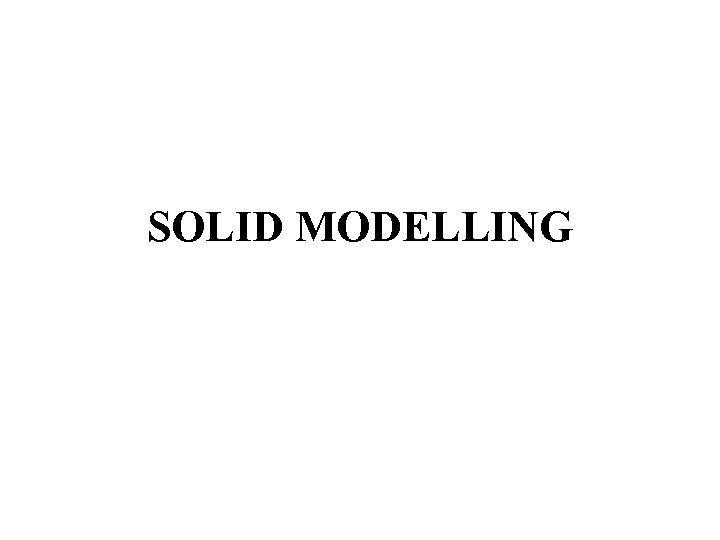SOLID MODELLING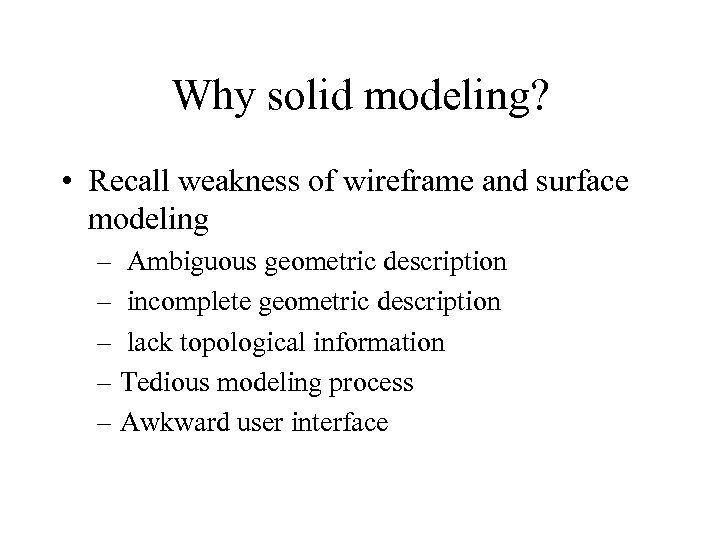Why solid modeling? • Recall weakness of wireframe and surface modeling – Ambiguous geometric description – incomplete geometric description – lack topological information – Tedious modeling process – Awkward user interface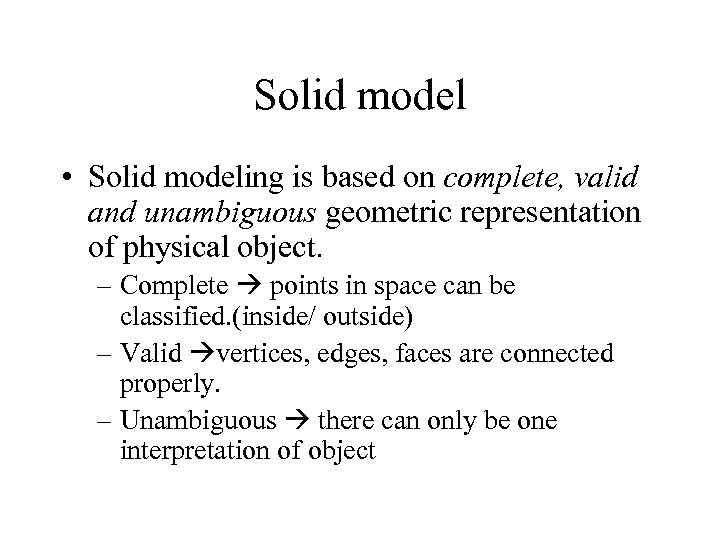Solid model • Solid modeling is based on complete, valid and unambiguous geometric representation of physical object. – Complete points in space can be classified. (inside/ outside) – Valid vertices, edges, faces are connected properly. – Unambiguous there can only be one interpretation of object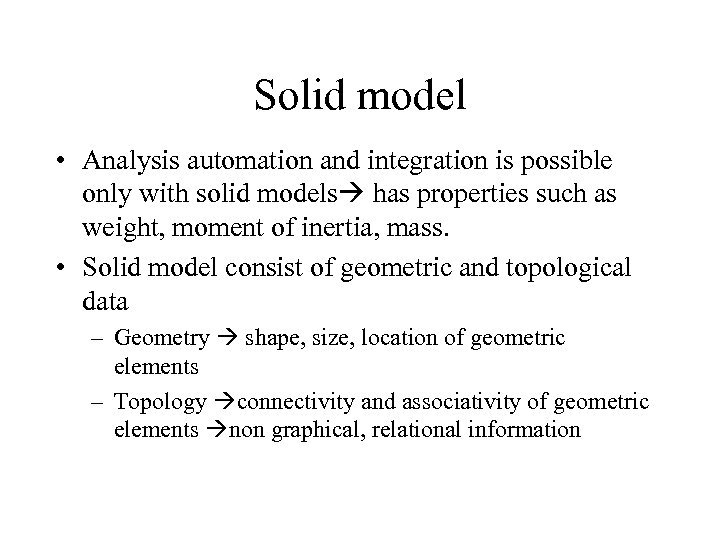Solid model • Analysis automation and integration is possible only with solid models has properties such as weight, moment of inertia, mass. • Solid model consist of geometric and topological data – Geometry shape, size, location of geometric elements – Topology connectivity and associativity of geometric elements non graphical, relational information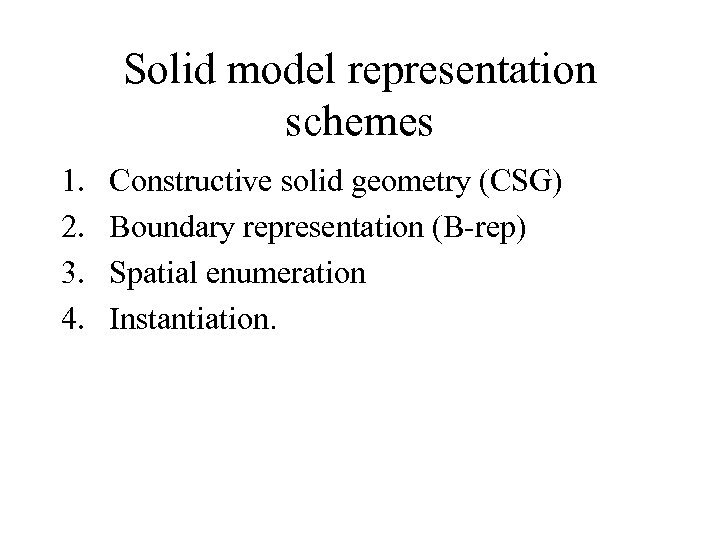Solid model representation schemes 1. 2. 3. 4. Constructive solid geometry (CSG) Boundary representation (B-rep) Spatial enumeration Instantiation.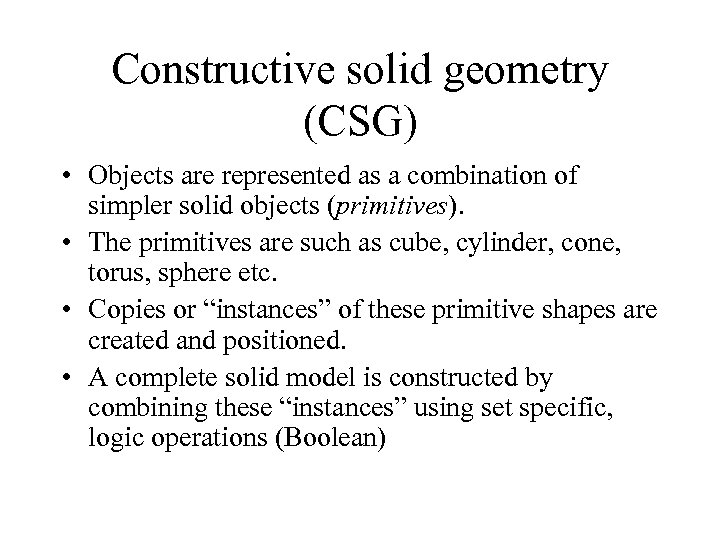Constructive solid geometry (CSG) • Objects are represented as a combination of simpler solid objects (primitives). • The primitives are such as cube, cylinder, cone, torus, sphere etc. • Copies or “instances” of these primitive shapes are created and positioned. • A complete solid model is constructed by combining these “instances” using set specific, logic operations (Boolean)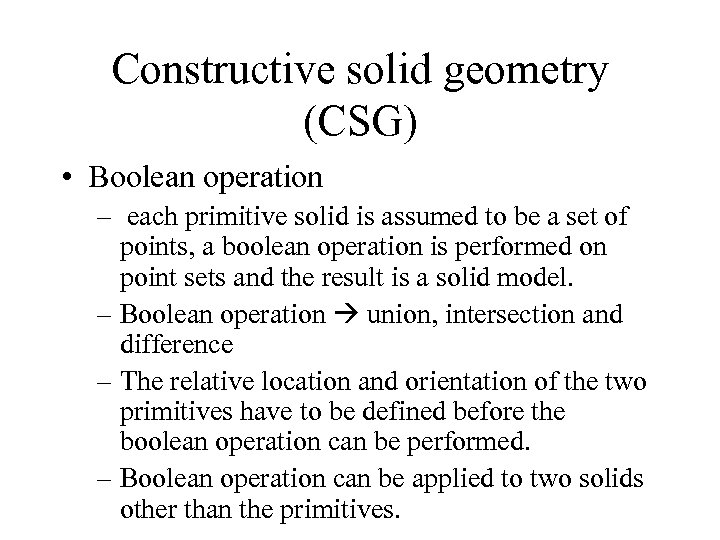Constructive solid geometry (CSG) • Boolean operation – each primitive solid is assumed to be a set of points, a boolean operation is performed on point sets and the result is a solid model. – Boolean operation union, intersection and difference – The relative location and orientation of the two primitives have to be defined before the boolean operation can be performed. – Boolean operation can be applied to two solids other than the primitives.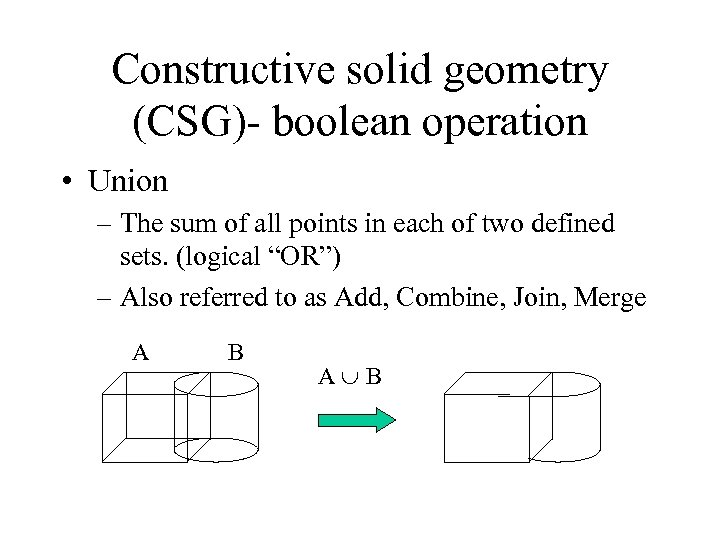Constructive solid geometry (CSG)- boolean operation • Union – The sum of all points in each of two defined sets. (logical “OR”) – Also referred to as Add, Combine, Join, Merge A B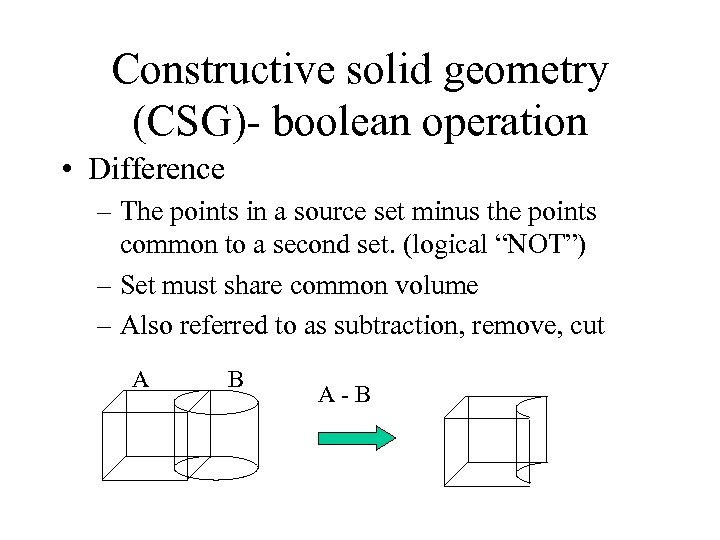Constructive solid geometry (CSG)- boolean operation • Difference – The points in a source set minus the points common to a second set. (logical “NOT”) – Set must share common volume – Also referred to as subtraction, remove, cut A B A-B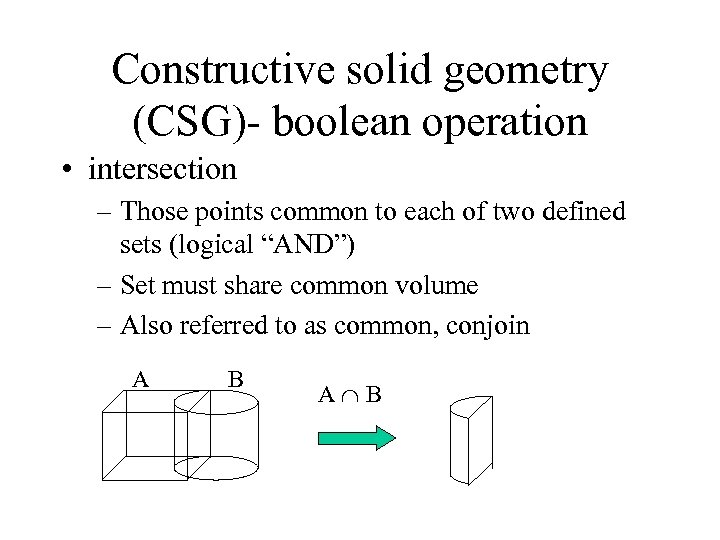Constructive solid geometry (CSG)- boolean operation • intersection – Those points common to each of two defined sets (logical “AND”) – Set must share common volume – Also referred to as common, conjoin A B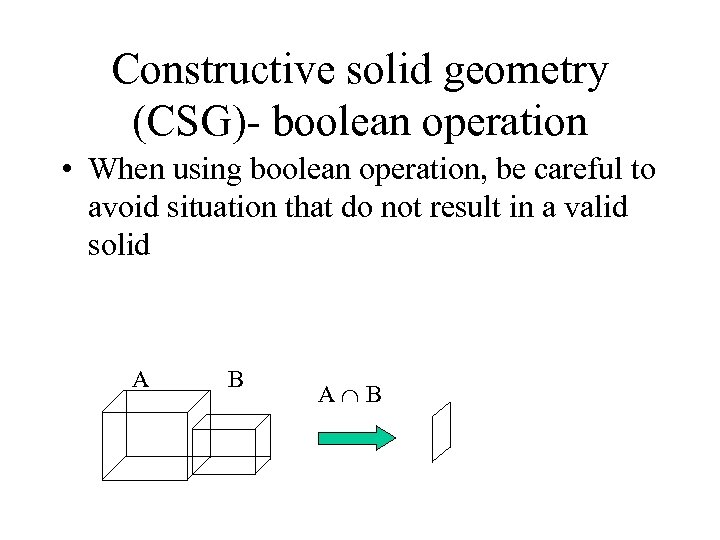Constructive solid geometry (CSG)- boolean operation • When using boolean operation, be careful to avoid situation that do not result in a valid solid A B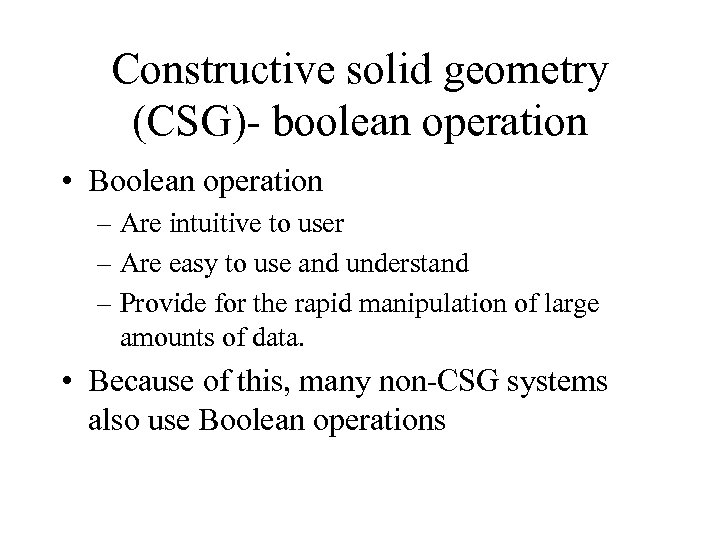Constructive solid geometry (CSG)- boolean operation • Boolean operation – Are intuitive to user – Are easy to use and understand – Provide for the rapid manipulation of large amounts of data. • Because of this, many non-CSG systems also use Boolean operations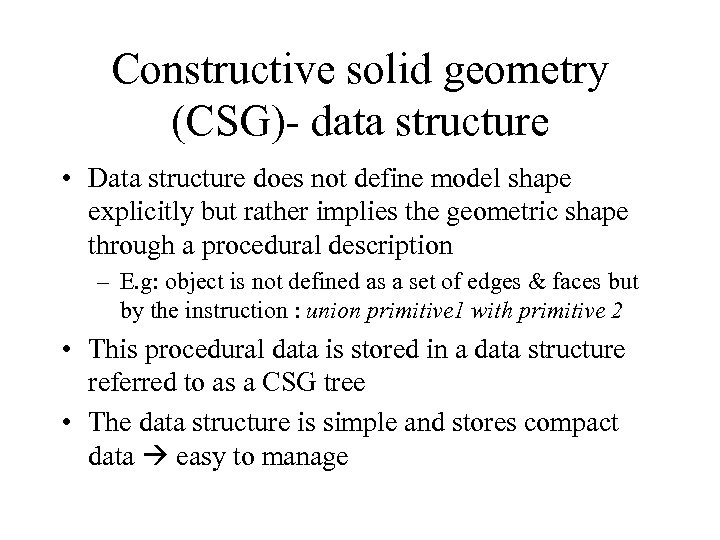Constructive solid geometry (CSG)- data structure • Data structure does not define model shape explicitly but rather implies the geometric shape through a procedural description – E. g: object is not defined as a set of edges & faces but by the instruction : union primitive 1 with primitive 2 • This procedural data is stored in a data structure referred to as a CSG tree • The data structure is simple and stores compact data easy to manage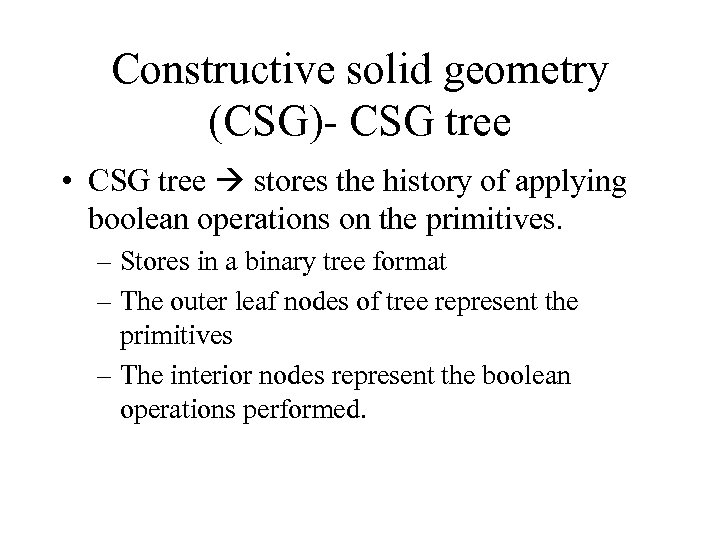Constructive solid geometry (CSG)- CSG tree • CSG tree stores the history of applying boolean operations on the primitives. – Stores in a binary tree format – The outer leaf nodes of tree represent the primitives – The interior nodes represent the boolean operations performed.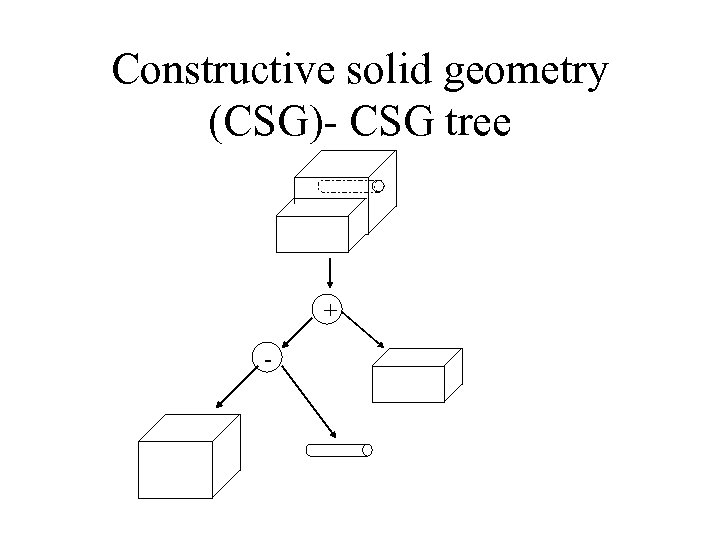Constructive solid geometry (CSG)- CSG tree + -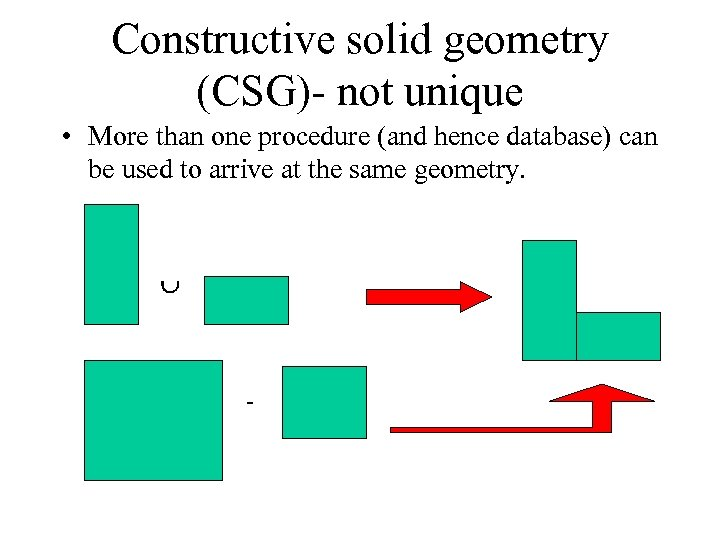Constructive solid geometry (CSG)- not unique • More than one procedure (and hence database) can be used to arrive at the same geometry. -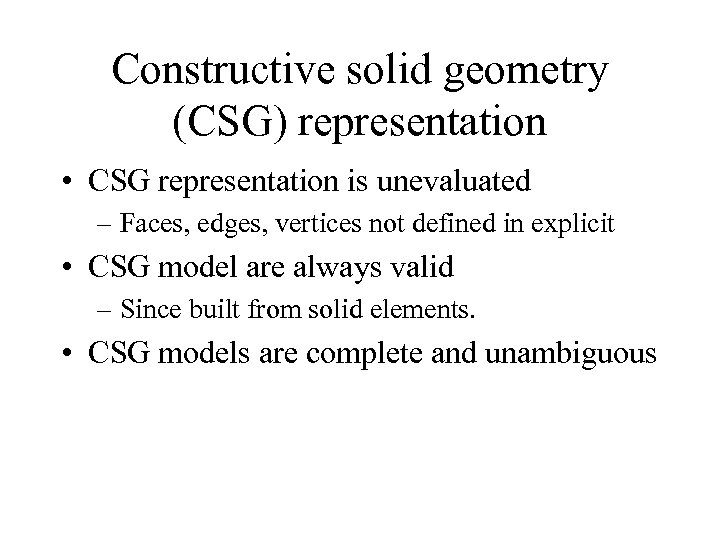Constructive solid geometry (CSG) representation • CSG representation is unevaluated – Faces, edges, vertices not defined in explicit • CSG model are always valid – Since built from solid elements. • CSG models are complete and unambiguous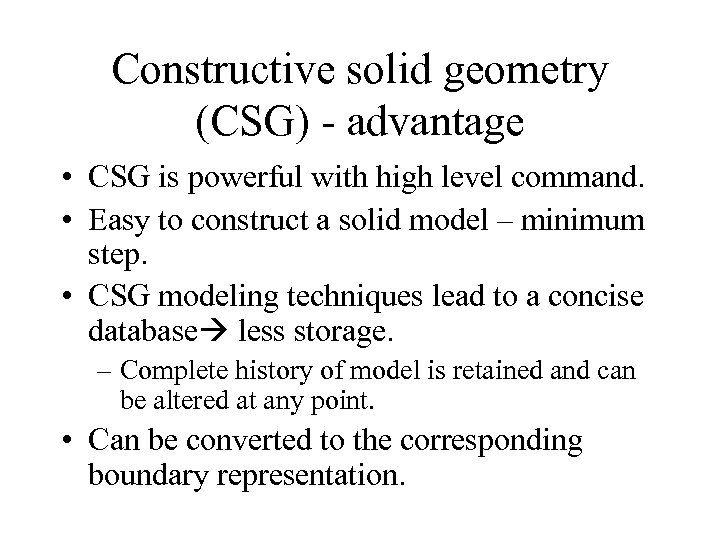Constructive solid geometry (CSG) - advantage • CSG is powerful with high level command. • Easy to construct a solid model – minimum step. • CSG modeling techniques lead to a concise database less storage. – Complete history of model is retained and can be altered at any point. • Can be converted to the corresponding boundary representation.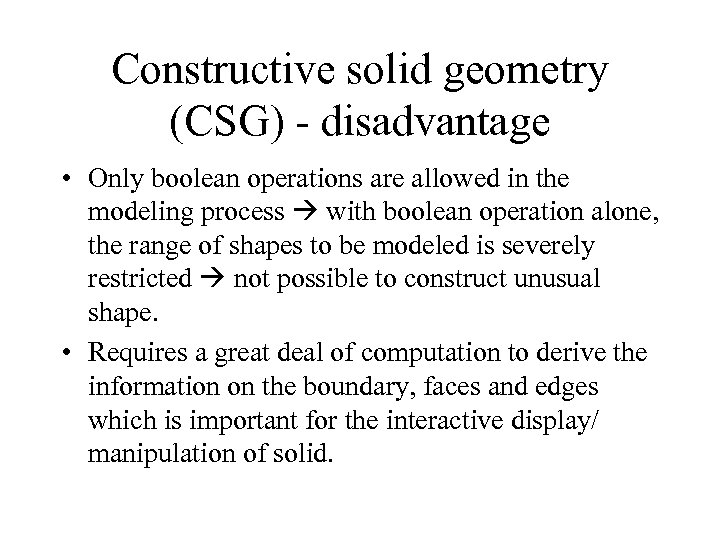Constructive solid geometry (CSG) - disadvantage • Only boolean operations are allowed in the modeling process with boolean operation alone, the range of shapes to be modeled is severely restricted not possible to construct unusual shape. • Requires a great deal of computation to derive the information on the boundary, faces and edges which is important for the interactive display/ manipulation of solid.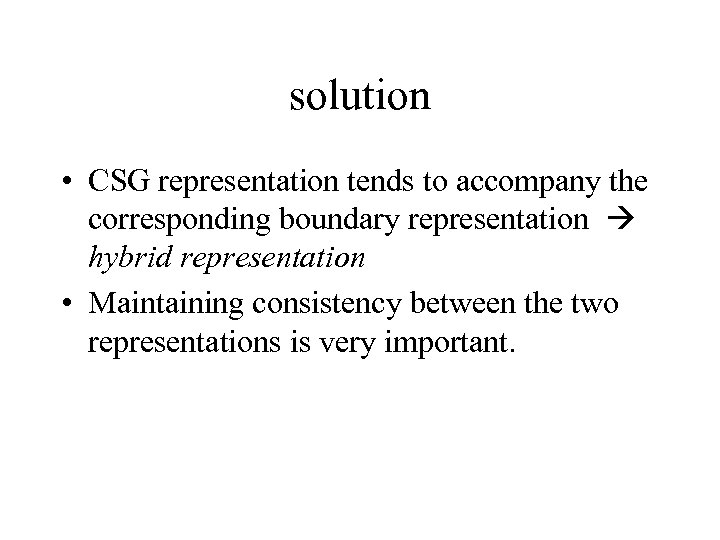solution • CSG representation tends to accompany the corresponding boundary representation hybrid representation • Maintaining consistency between the two representations is very important.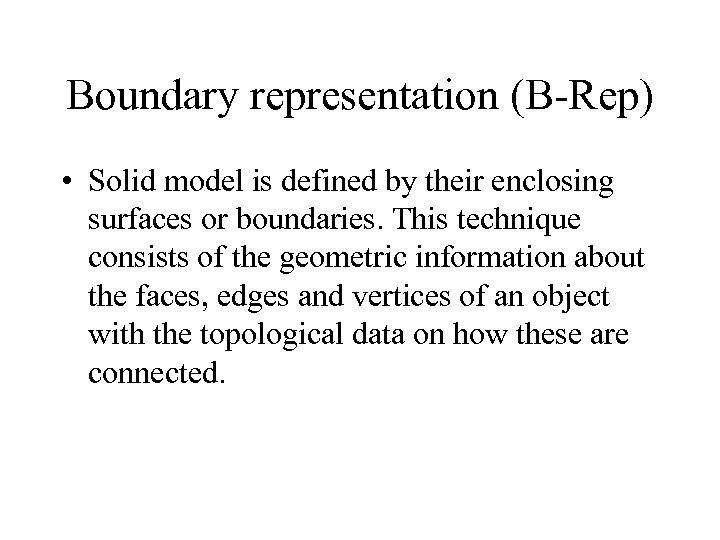Boundary representation (B-Rep) • Solid model is defined by their enclosing surfaces or boundaries. This technique consists of the geometric information about the faces, edges and vertices of an object with the topological data on how these are connected.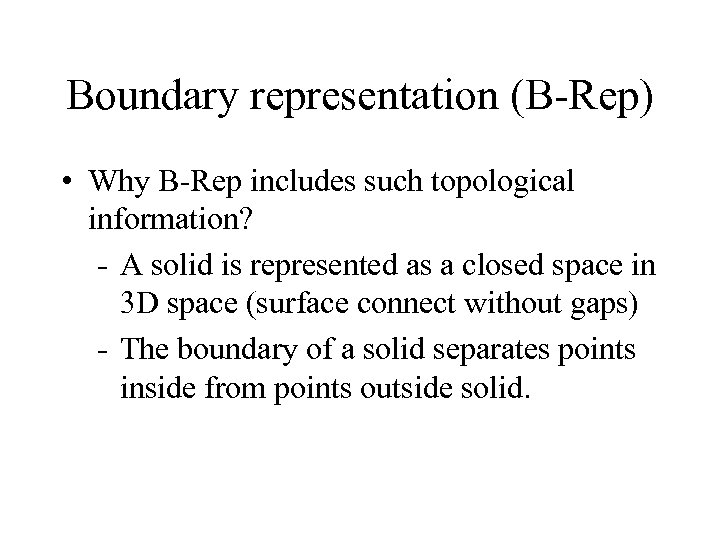Boundary representation (B-Rep) • Why B-Rep includes such topological information? - A solid is represented as a closed space in 3 D space (surface connect without gaps) - The boundary of a solid separates points inside from points outside solid.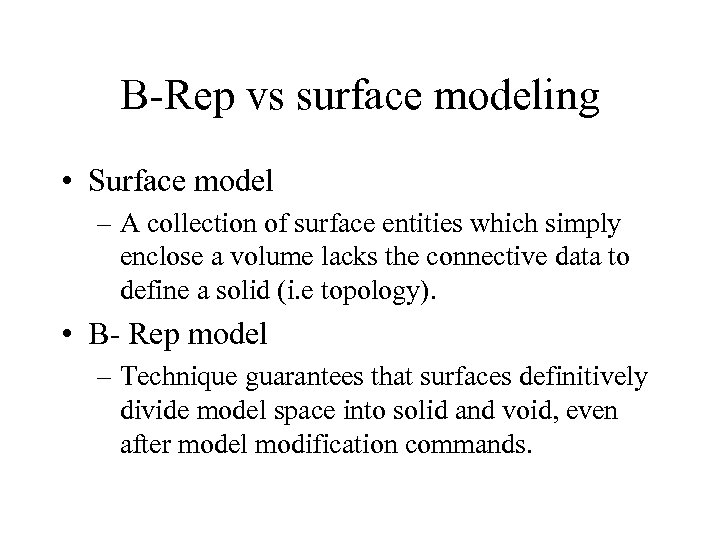B-Rep vs surface modeling • Surface model – A collection of surface entities which simply enclose a volume lacks the connective data to define a solid (i. e topology). • B- Rep model – Technique guarantees that surfaces definitively divide model space into solid and void, even after model modification commands.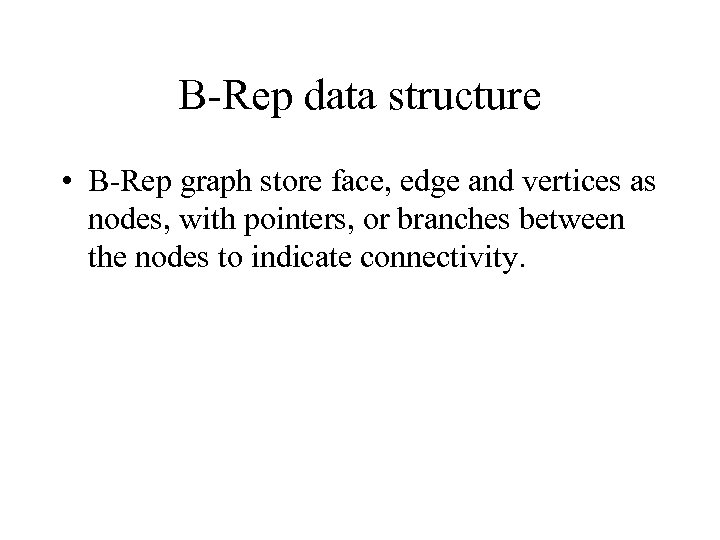B-Rep data structure • B-Rep graph store face, edge and vertices as nodes, with pointers, or branches between the nodes to indicate connectivity.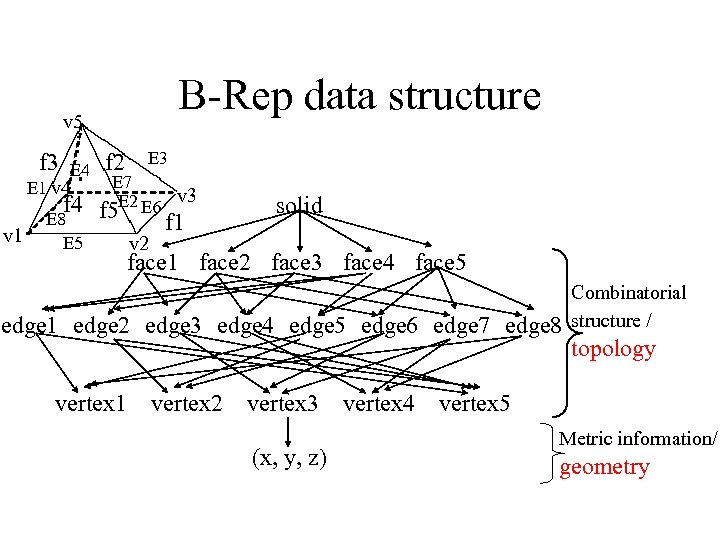B-Rep data structure v 5 f 3 E 4 E 1 v 4 v 1 E 3 f 2 E 7 E 2 E 6 v 3 f 4 f 5 E 8 E 5 v 2 f 1 solid face 1 face 2 face 3 face 4 face 5 edge 1 edge 2 edge 3 edge 4 edge 5 edge 6 edge 7 edge 8 vertex 1 vertex 2 vertex 3 (x, y, z) vertex 4 Combinatorial structure / topology vertex 5 Metric information/ geometryBoundary representation- validity • System must validate topology of created solid. • B-Rep has to fulfill certain conditions to disallow self-intersecting and open objects • This condition include – Each edge should adjoin exactly two faces and have a vertex at each end. – Vertices are geometrically described by point coordinates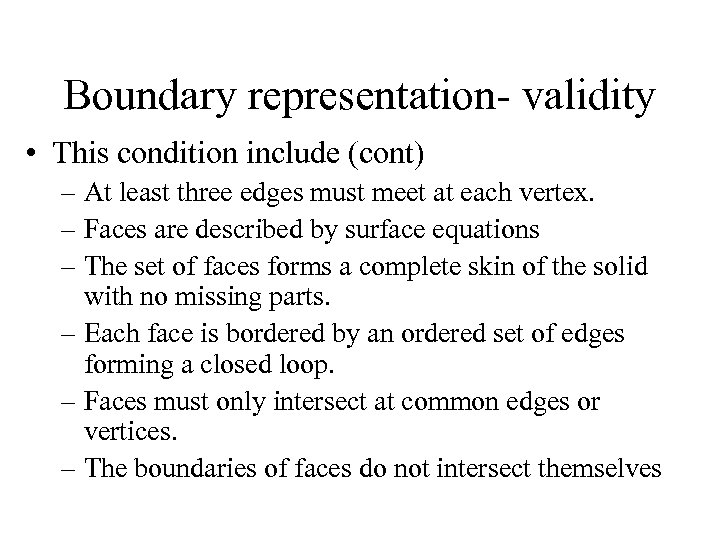Boundary representation- validity • This condition include (cont) – At least three edges must meet at each vertex. – Faces are described by surface equations – The set of faces forms a complete skin of the solid with no missing parts. – Each face is bordered by an ordered set of edges forming a closed loop. – Faces must only intersect at common edges or vertices. – The boundaries of faces do not intersect themselves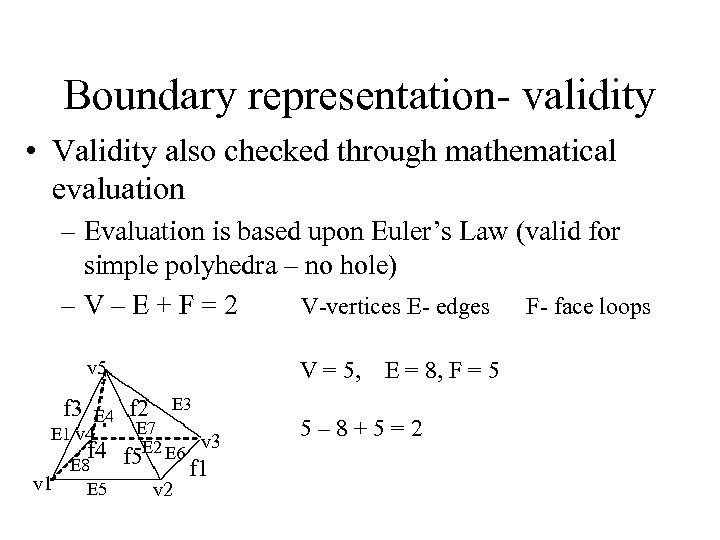Boundary representation- validity • Validity also checked through mathematical evaluation – Evaluation is based upon Euler’s Law (valid for simple polyhedra – no hole) –V–E+F=2 V-vertices E- edges F- face loops v 5 f 3 E 4 E 1 v 4 V = 5, f 2 E 3 E 7 E 2 E 6 v 3 f 4 f 5 E 8 v 1 E 5 E = 8, F = 5 v 2 f 1 5– 8+5=2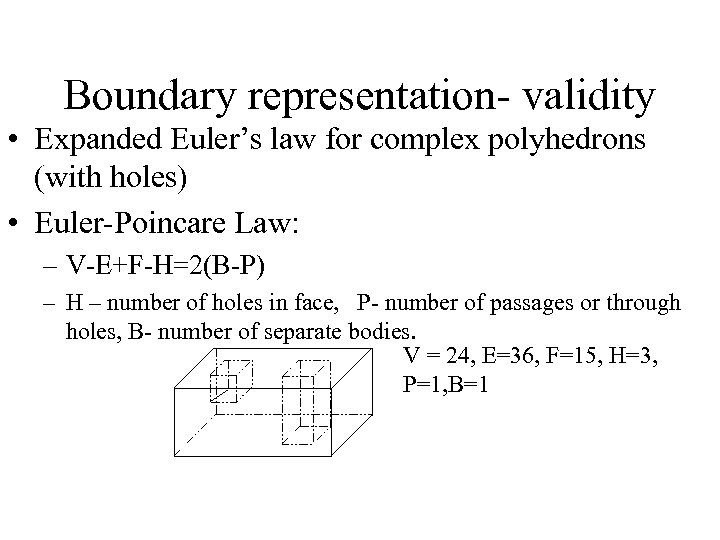Boundary representation- validity • Expanded Euler’s law for complex polyhedrons (with holes) • Euler-Poincare Law: – V-E+F-H=2(B-P) – H – number of holes in face, P- number of passages or through holes, B- number of separate bodies. V = 24, E=36, F=15, H=3, P=1, B=1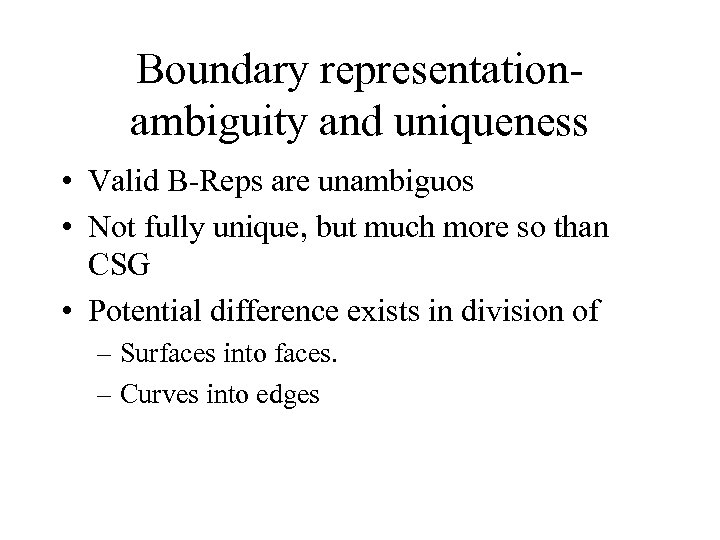Boundary representationambiguity and uniqueness • Valid B-Reps are unambiguos • Not fully unique, but much more so than CSG • Potential difference exists in division of – Surfaces into faces. – Curves into edges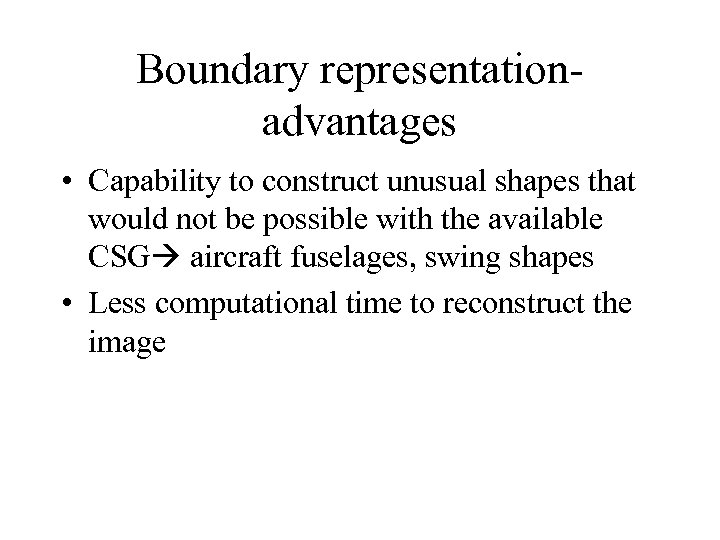Boundary representationadvantages • Capability to construct unusual shapes that would not be possible with the available CSG aircraft fuselages, swing shapes • Less computational time to reconstruct the image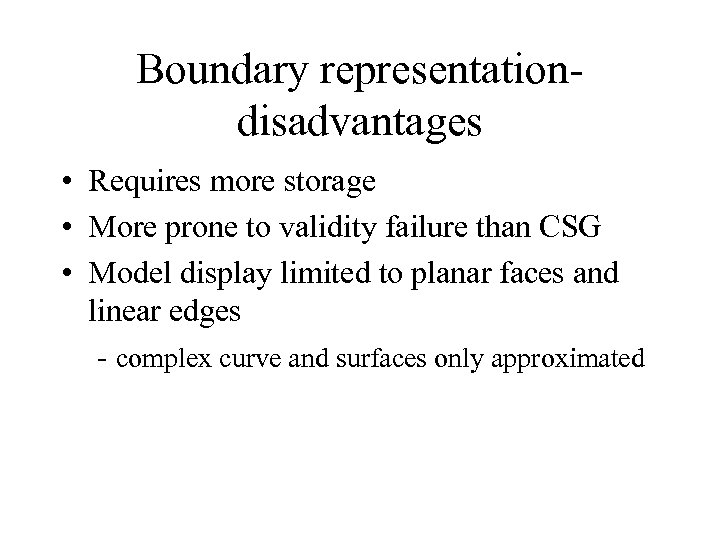Boundary representationdisadvantages • Requires more storage • More prone to validity failure than CSG • Model display limited to planar faces and linear edges - complex curve and surfaces only approximated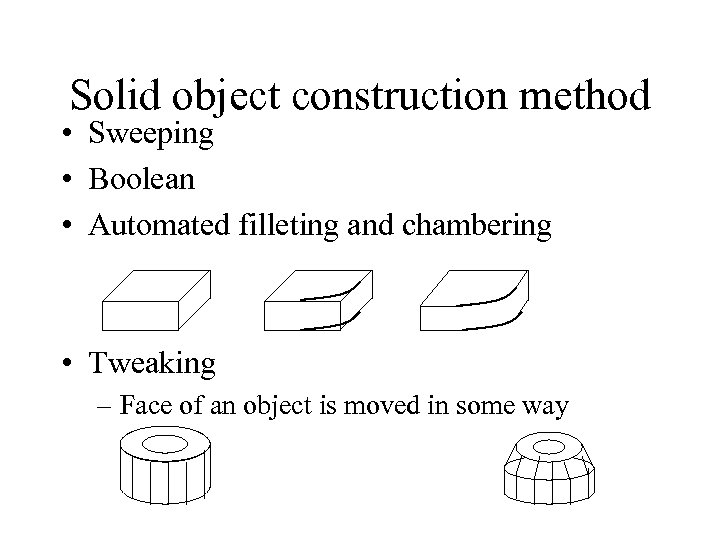Solid object construction method • Sweeping • Boolean • Automated filleting and chambering • Tweaking – Face of an object is moved in some way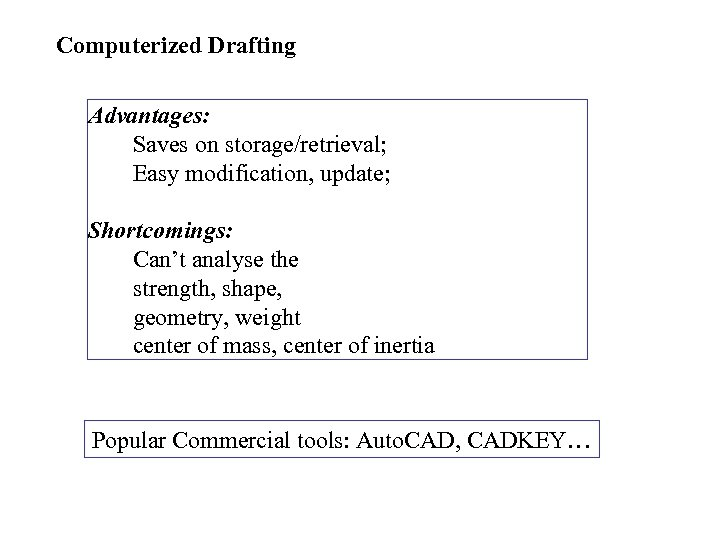Computerized Drafting Advantages: Saves on storage/retrieval; Easy modification, update; Shortcomings: Can’t analyse the strength, shape, geometry, weight center of mass, center of inertia Popular Commercial tools: Auto. CAD, CADKEY…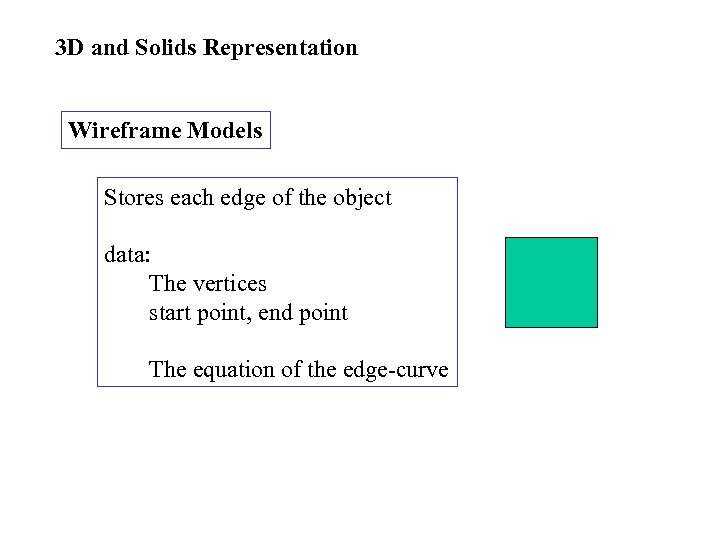3 D and Solids Representation Wireframe Models Stores each edge of the object data: The vertices start point, end point The equation of the edge-curve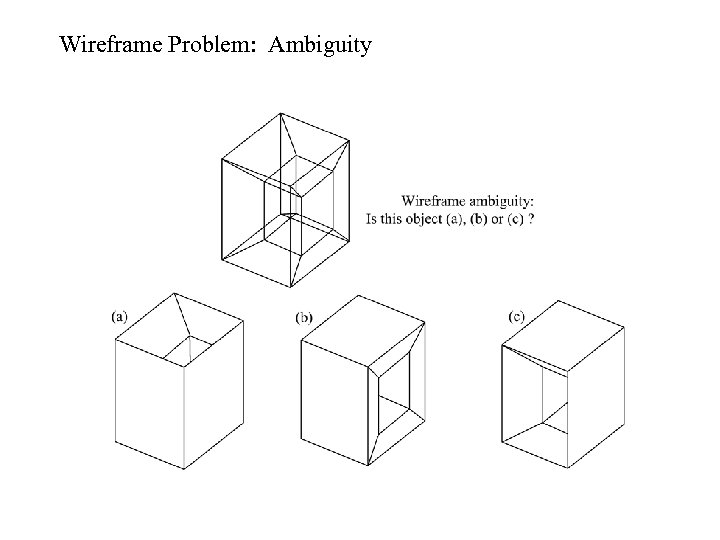Wireframe Problem: Ambiguity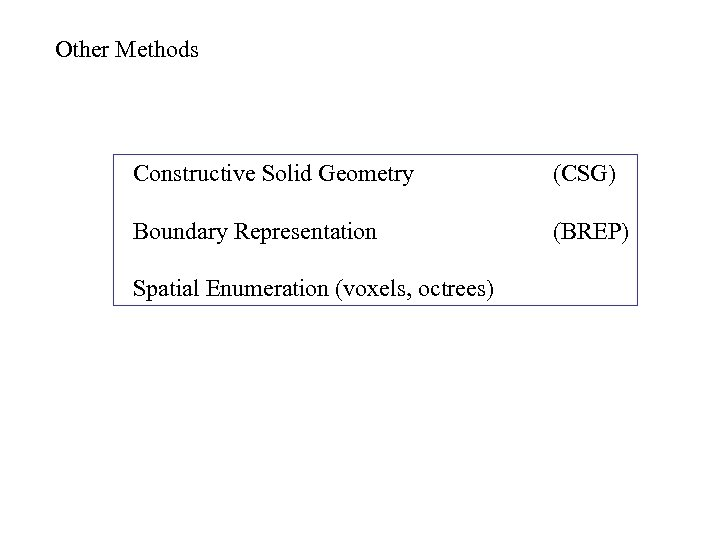Other Methods Constructive Solid Geometry (CSG) Boundary Representation (BREP) Spatial Enumeration (voxels, octrees)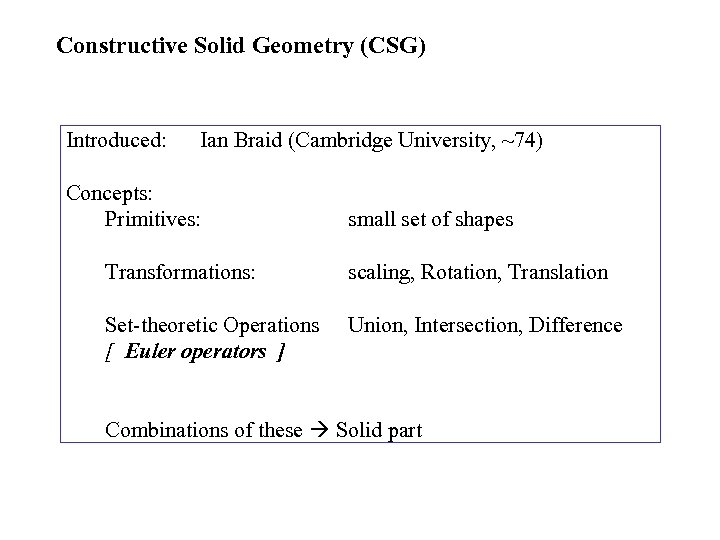Constructive Solid Geometry (CSG) Introduced: Ian Braid (Cambridge University, ~74) Concepts: Primitives: small set of shapes Transformations: scaling, Rotation, Translation Set-theoretic Operations [ Euler operators ] Union, Intersection, Difference Combinations of these Solid part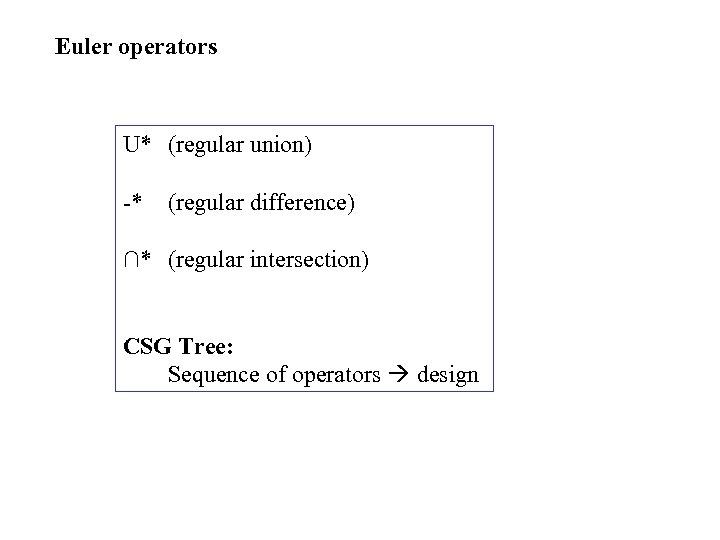Euler operators U* (regular union) -* (regular difference) ∩* (regular intersection) CSG Tree: Sequence of operators design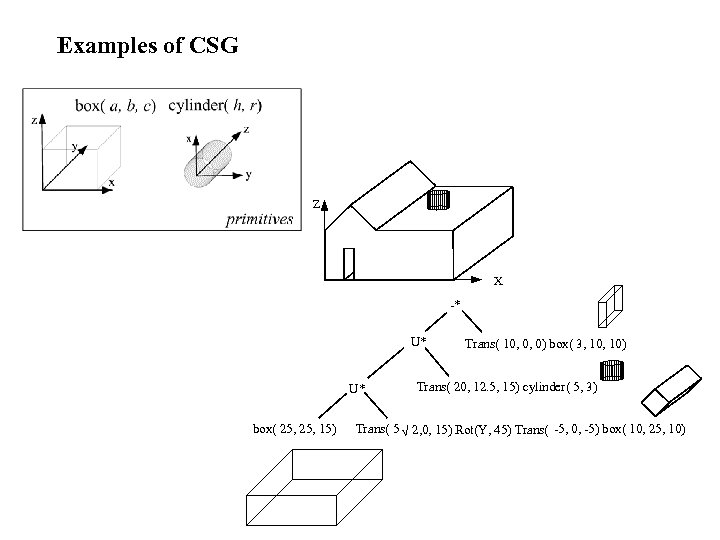Examples of CSG Z X -* U* U* box( 25, 15) Trans( 10, 0, 0) box( 3, 10) Trans( 20, 12. 5, 15) cylinder( 5, 3) Trans( 5 2, 0, 15) Rot(Y, 45) Trans( -5, 0, -5) box( 10, 25, 10)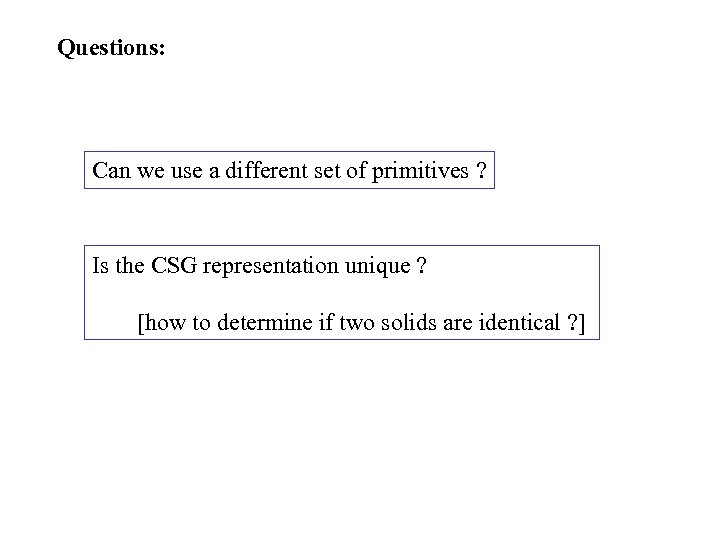Questions: Can we use a different set of primitives ? Is the CSG representation unique ? [how to determine if two solids are identical ? ]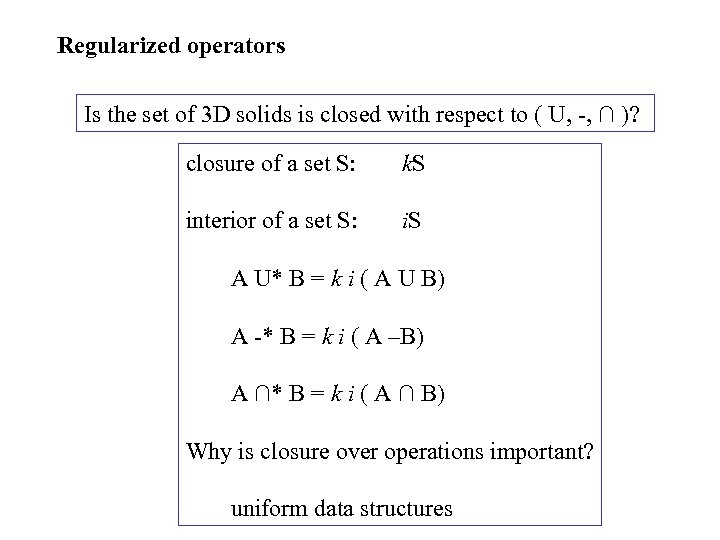Regularized operators Is the set of 3 D solids is closed with respect to ( U, -, ∩ )? closure of a set S: k. S interior of a set S: i. S A U* B = k i ( A U B) A -* B = k i ( A –B) A ∩* B = k i ( A ∩ B) Why is closure over operations important? uniform data structures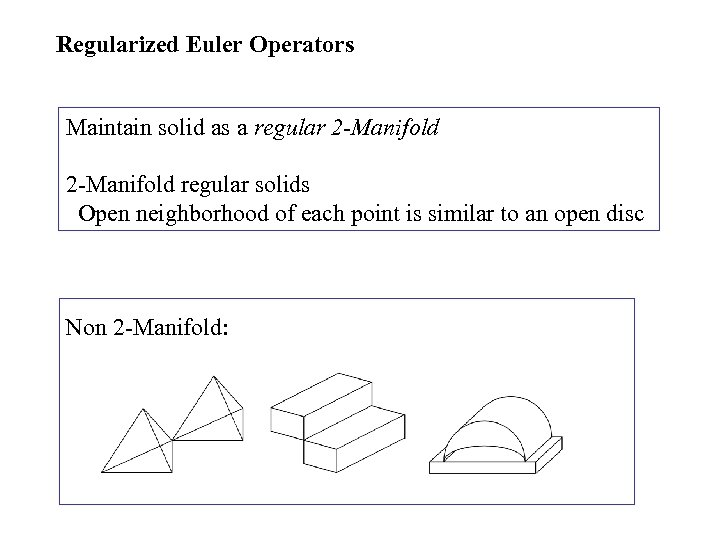Regularized Euler Operators Maintain solid as a regular 2 -Manifold regular solids Open neighborhood of each point is similar to an open disc Non 2 -Manifold: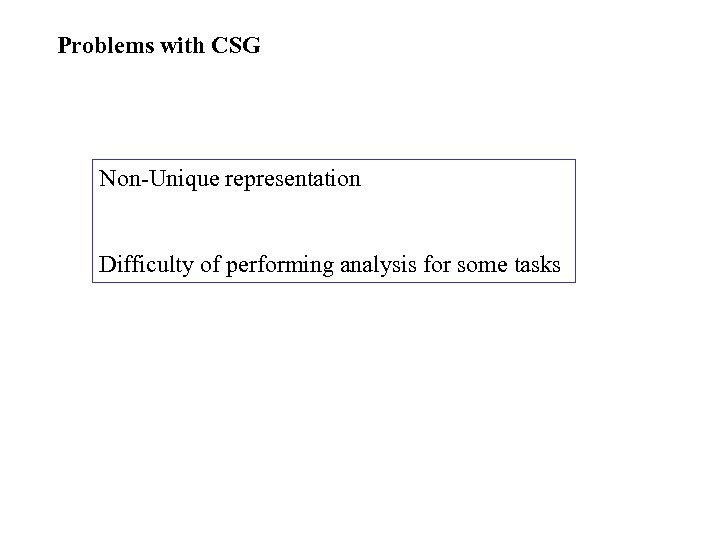Problems with CSG Non-Unique representation Difficulty of performing analysis for some tasks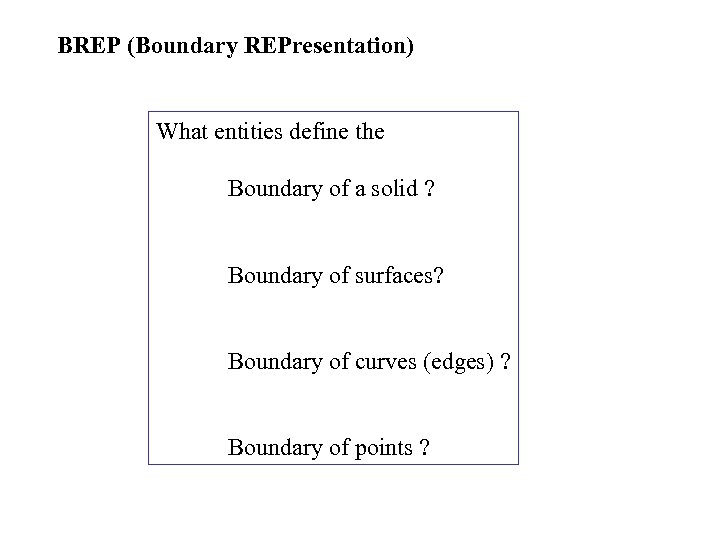BREP (Boundary REPresentation) What entities define the Boundary of a solid ? Boundary of surfaces? Boundary of curves (edges) ? Boundary of points ?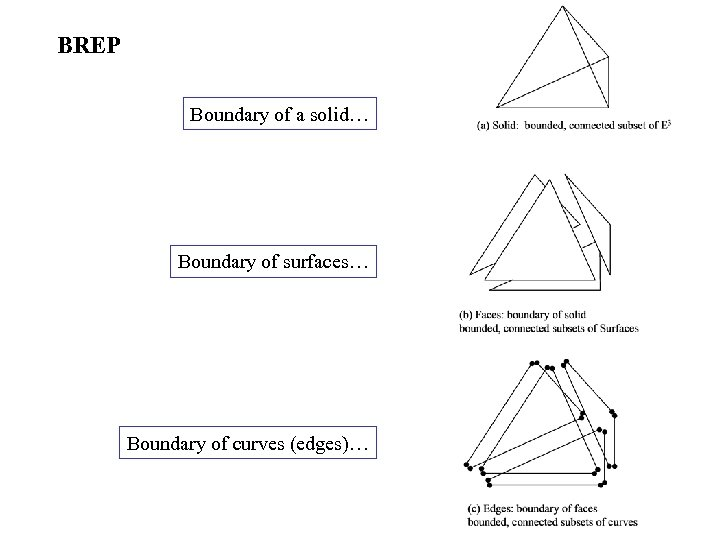BREP Boundary of a solid… Boundary of surfaces… Boundary of curves (edges)…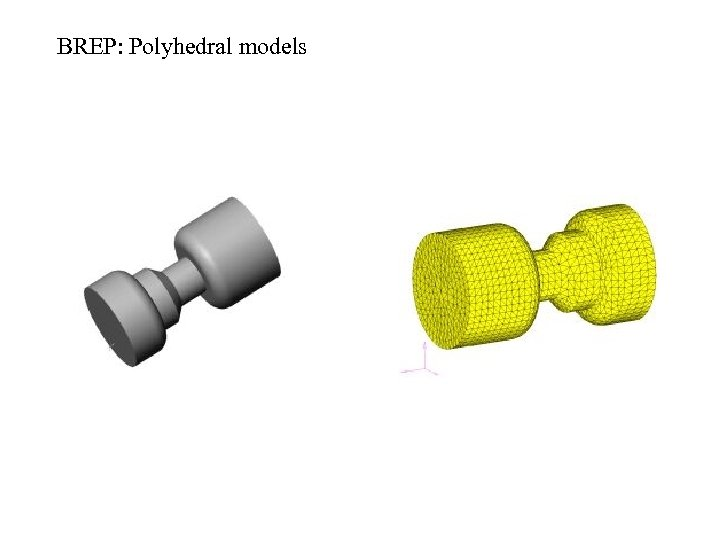BREP: Polyhedral models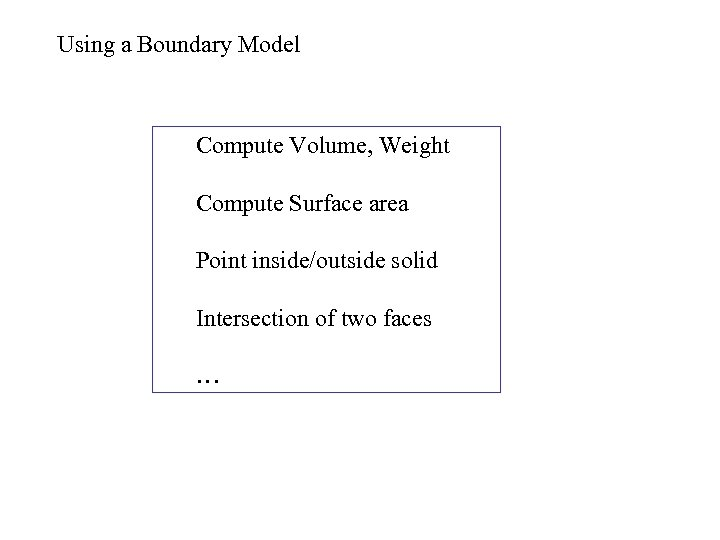Using a Boundary Model Compute Volume, Weight Compute Surface area Point inside/outside solid Intersection of two faces …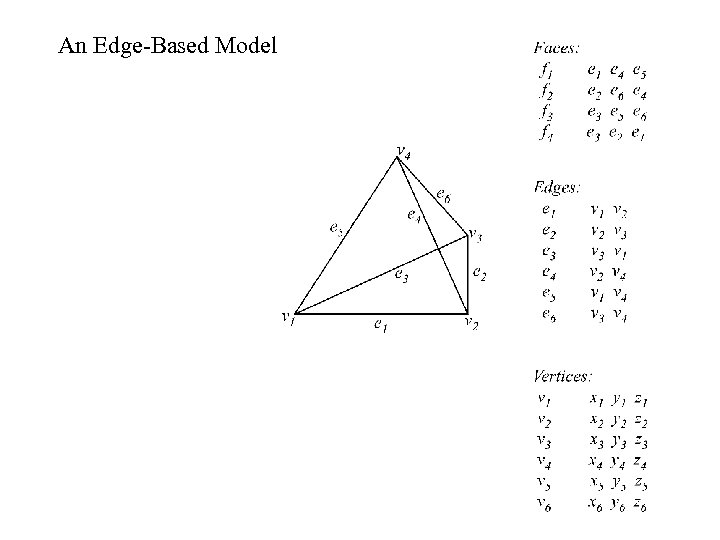An Edge-Based Model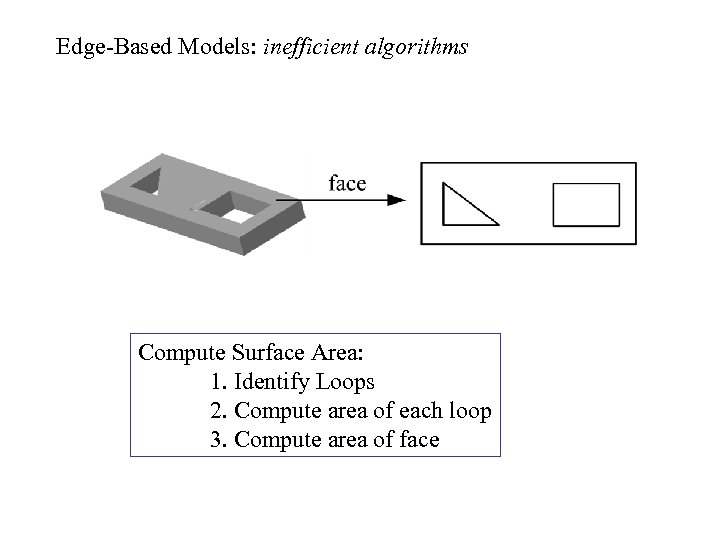Edge-Based Models: inefficient algorithms Compute Surface Area: 1. Identify Loops 2. Compute area of each loop 3. Compute area of face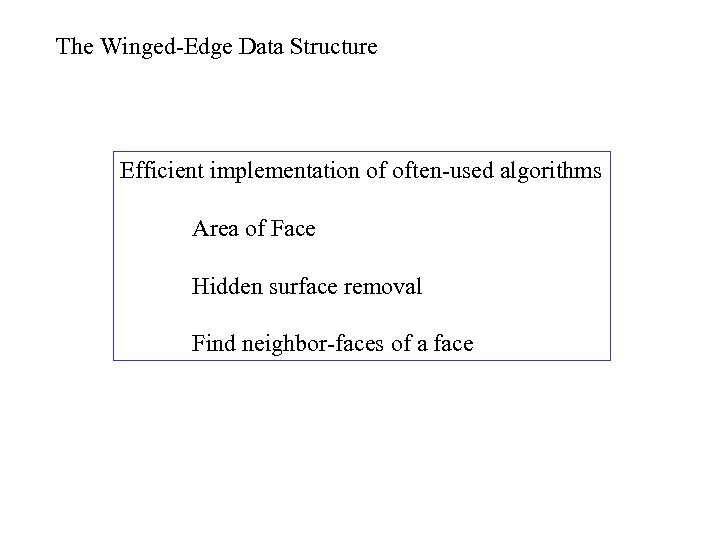The Winged-Edge Data Structure Efficient implementation of often-used algorithms Area of Face Hidden surface removal Find neighbor-faces of a face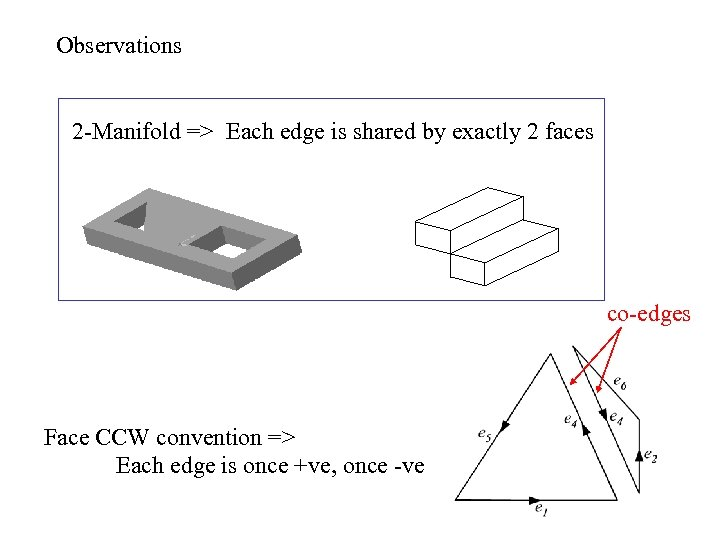Observations 2 -Manifold => Each edge is shared by exactly 2 faces co-edges Face CCW convention => Each edge is once +ve, once -ve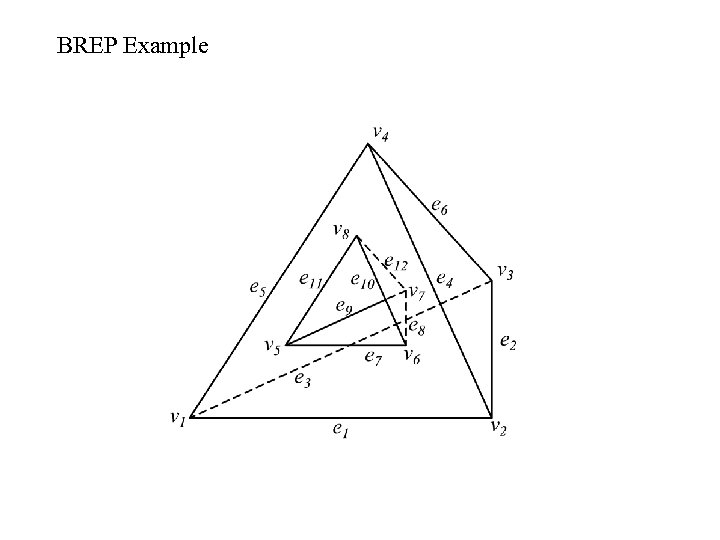BREP Example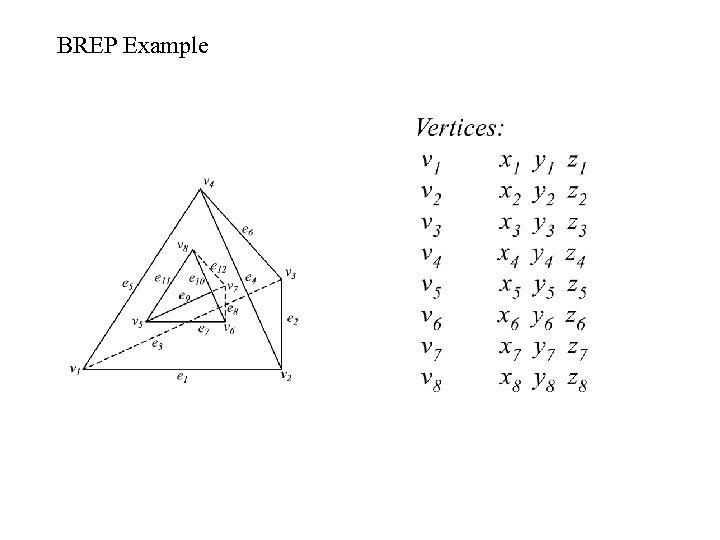BREP Example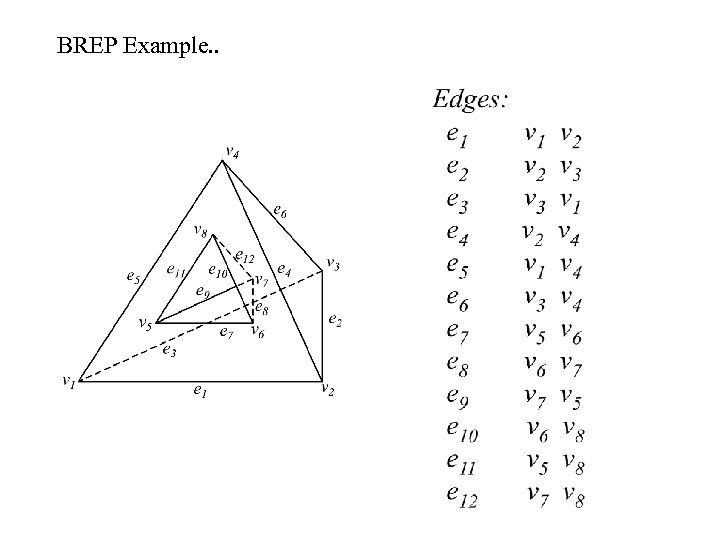BREP Example. .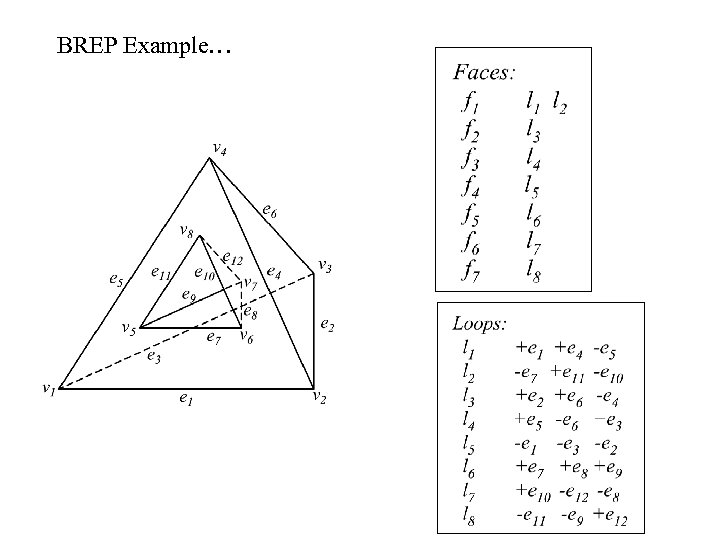BREP Example…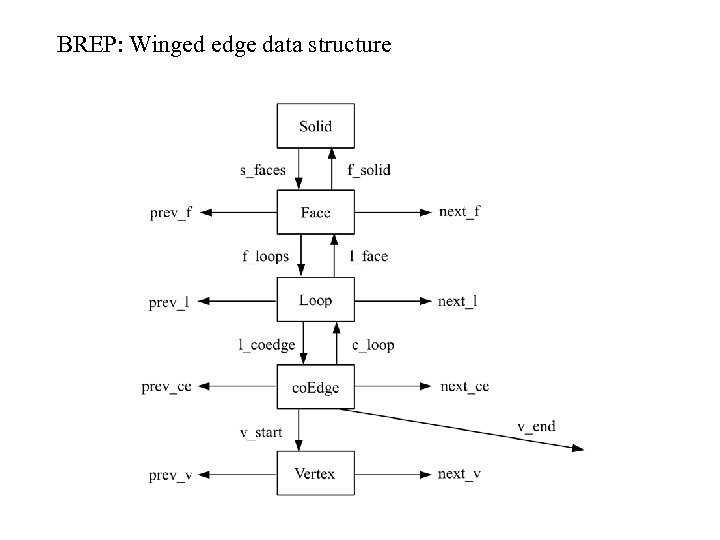BREP: Winged edge data structure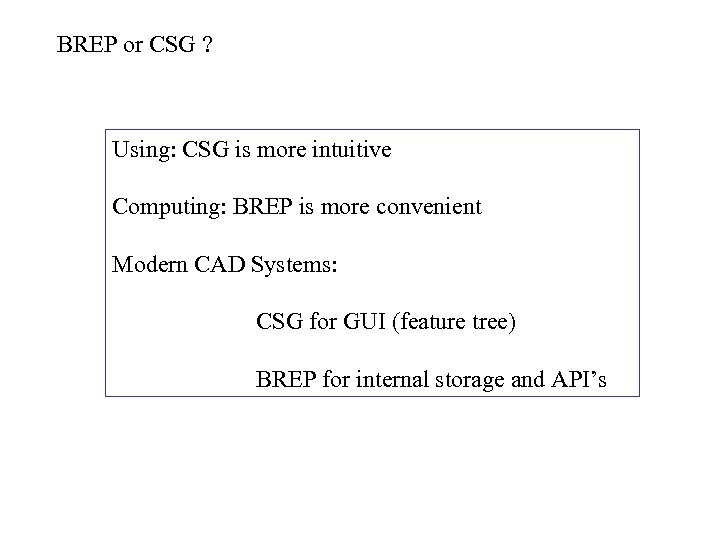BREP or CSG ? Using: CSG is more intuitive Computing: BREP is more convenient Modern CAD Systems: CSG for GUI (feature tree) BREP for internal storage and API’s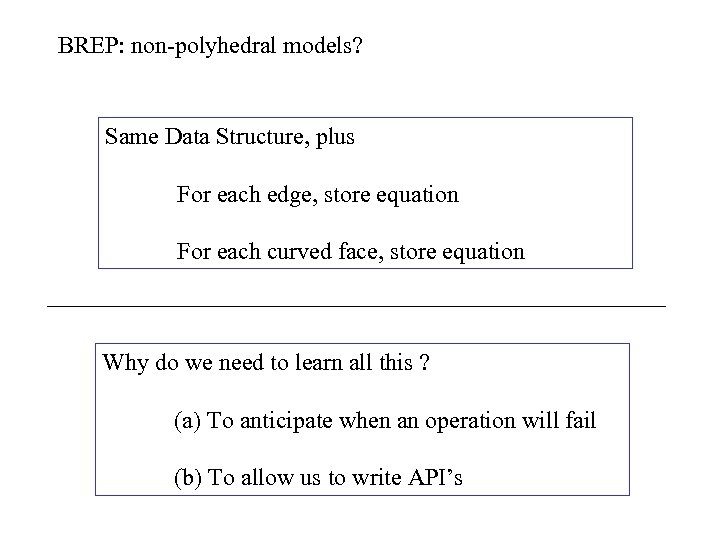BREP: non-polyhedral models? Same Data Structure, plus For each edge, store equation For each curved face, store equation Why do we need to learn all this ? (a) To anticipate when an operation will fail (b) To allow us to write API’s